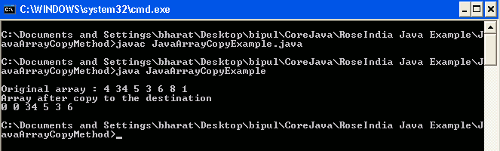Tutorials

# Java array copy example

In this section we will discuss about the arrayCopy() in Java.

In this section we will discuss about the arrayCopy() in Java.

## Java array copy example

In this section we will discuss about the arrayCopy() in Java.

arrayCopy() is a method of System class which copies the given length of element started from the specified position from the source array to the given position into the destination array. This method copies the element of one dimensional array. NullPointerException is thrown in case either source, destination or both are null. An ArrayStoreException can be thrown in case of either source, destination or both arguments are not an array, source and destination arguments refers to different primitive types arrays. An IndexOutOfBoundsException is thrown in case of either source position, destination position, length, is negative, source array length is less than the srcPos+length, destination array length is less than destPos+length.

Syntax :

```public static void arraycopy(Object src, int srcPos, Object dest, int destPos, int length)
```

Example

Here I am giving a simple example which will demonstrate you about how an specified length of source array beginning from the given position can be copied to the destination array at the specified position. In this example I have created a Java class named JavaArrayCopyExample.java. This class contains array of integer elements which elements will be copied to the another array. To copy the elements of an existing array I have created a new empty integer array with the specified size '6'. Then I used the arrayCopy() method to copy the elements to the specified array. Since array indexing is started from the 0 (zero) so the 0 will be the first position to store the element i.e. first position at index 0, second position at index 1 and so on. In this example I have given the src position =1 i.e. copying of elements will be started from the second position from original array and the destination position is given to 2, i.e. elements will be started to store at third position of destination array.

Source Code

JavaArrayCopyExample.java

```public class JavaArrayCopyExample {

public static void main(String args[])
{
int[] cpyFrom = {4,34,5,3,6,8,1};
int[] cpyTo = new int;
System.out.println();
System.out.print("Original array : ");
for(int i = 0; i<cpyFrom.length; i++)
{
System.out.print(cpyFrom[i]+" ");
}
System.out.println();
System.arraycopy(cpyFrom, 1, cpyTo, 2, cpyTo.length-2);
System.out.println("Array after copy " +
"to the destination");
for(int i=0; i<cpyTo.length; i++)
{
System.out.print(cpyTo[i]+" ");
}
System.out.println();
}
}
```

Output

When you will execute the above example you will get the output as follows :